Question

# Write and test a C program that uses this fact to estimate π as follows 1....

Write and test a C program that uses this fact to estimate π as follows
1. Enter N, set C to zero
2. Generate N random pairs of integers (A,B) and if A and B are co-prime then add 1 to C
3. Print the estimate of pi = sqrt ( (6*N)/C )

Ans:-

CODE

#include<stdio.h>
#include<stdlib.h>
#include<time.h>
#include<math.h>
int iscoprime(int a, int b);
int main()
{
int n,c=0;
int a,b;
float pi;
printf("Enter the value of n: ");
scanf("%d",&n);
//printf("Enter any number for randomization ");
srand(time(0));
for(int i=0;i<n;i++)
{
a=rand()%100;
b=rand()%100;
printf("number %d and %d are: ",a,b);
if(iscoprime(a,b)==1)
{

c=c+1;
printf(" coprime ");
}
else printf(" not coprime ");
}
pi = sqrt ( (6*n)/c );
printf("The estimate of pi is %f",pi);

return 0;
}

int iscoprime(int a, int b) {

int gcd = 1;
int i;

for(i = 1; i < a || i < b ; ++i) {

if( (a % i == 0) && (b % i == 0)) {

gcd = i;
}
}

if ( gcd == 1 )
return 1;
else
return 0;
}

OUTPUT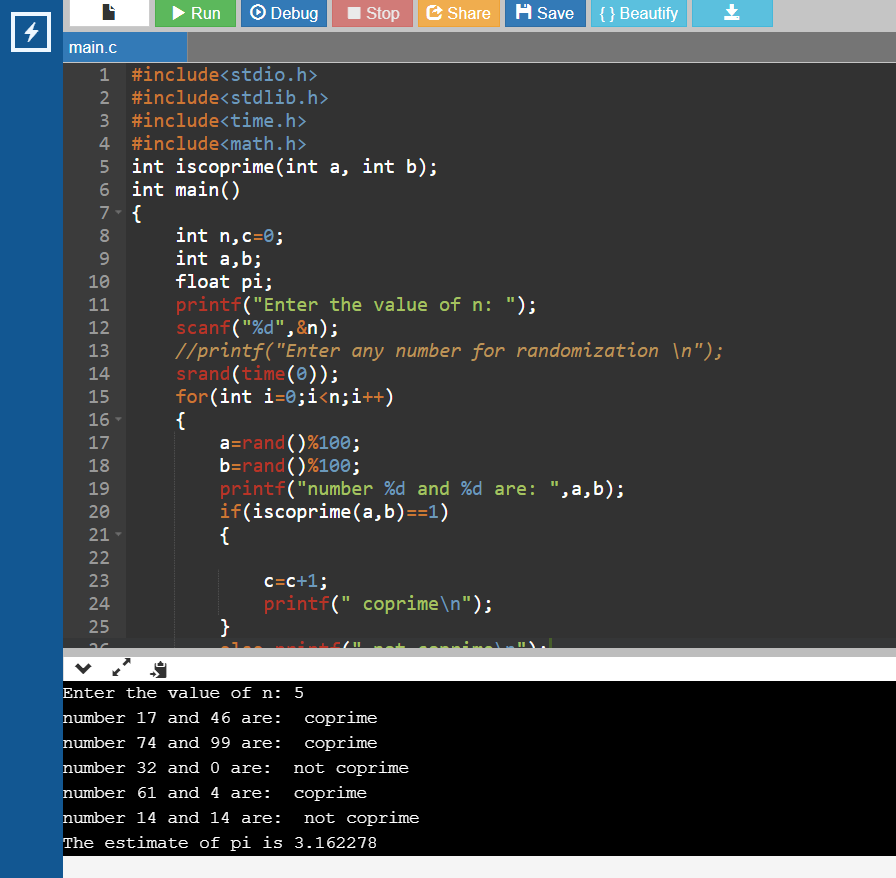P.S. - If you find my answer useful, please take a second to give it a THUMBS UP.

#### Earn Coins

Coins can be redeemed for fabulous gifts.

Similar Homework Help Questions
• ### Write a Java program that uses the Monte Carlo method to estimate the value of PI....

Write a Java program that uses the Monte Carlo method to estimate the value of PI. This method uses the unit circle inscribed in a square with sides of length 2 and random numbers to perform the estimation. The estimation works as follows: • Two random numbers are generated during each iteration of a loop. • The random numbers are each in the range of -1 to 1. One random number is the x-coordinate and the other is the y-coordinate....

• ### C++ Write a program that prompts the user to enter integers or a sentinel to stop....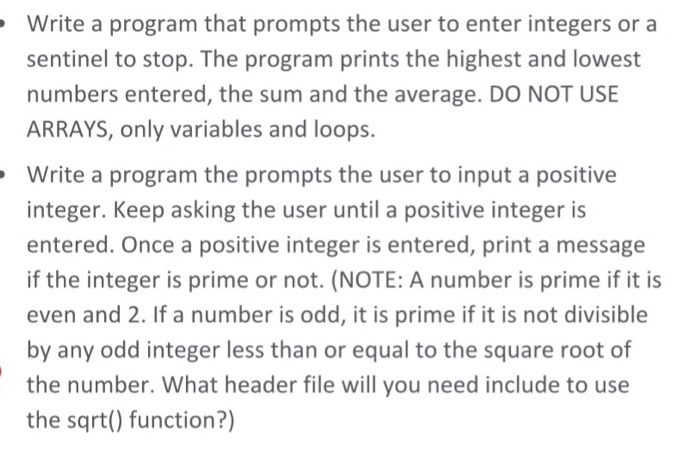C++ Write a program that prompts the user to enter integers or a sentinel to stop. The program prints the highest and lowest numbers entered, the sum and the average. DO NOT USE ARRAYS, only variables and loops. Write a program the prompts the user to input a positive integer. Keep asking the user until a positive integer is entered. Once a positive integer is entered, print a message if the integer is prime or not. (NOTE: A number is...

• ### PLease IN C not in C++ or JAVA, Lab3. Write a computer program in C which...

PLease IN C not in C++ or JAVA, Lab3. Write a computer program in C which will simulate a calculator. Your calculator needs to support the five basic operations (addition, subtraction, multiplication, division and modulus) plus primality testing (natural number is prime if it has no non-trivial divisors). : Rewrite your lab 3 calculator program using functions. Each mathematical operation in the menu should be represented by a separate function. In addition to all operations your calculator had to support...

• ### Write a C++ program that computes the following series: sum = 1/firstPrime + 2/secondPrime+…..+1/nthPrime Your program...

Write a C++ program that computes the following series: sum = 1/firstPrime + 2/secondPrime+…..+1/nthPrime Your program should prompt the user to enter a number n. The program will compute and display sum based on the series defined above. firstPrime: is 2 secondPrime: the first prime number after 2 thirdPrime: the third prime number …. nth prime: the nth prime number Your program must be organized as follows: int main() { //prompt the user to enter n //read n from the...

• ### c++ 2. Write a function to test primality Use the definition of prime number to guide...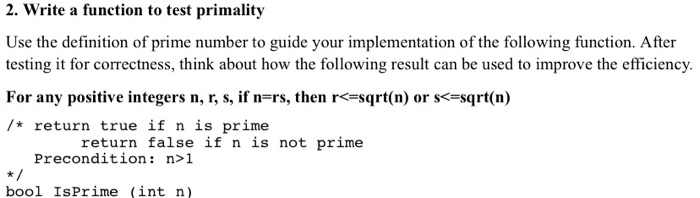c++ 2. Write a function to test primality Use the definition of prime number to guide your implementation of the following function. After testing it for correctness, think about how the following result can be used to improve the efficiency. For any positive integers n, r, s, if n=rs, then r<=sqrt(n) or s<=sqrt(n) /* return true if n is prime return false if n is not prime Precondition: n>1 bool IsPrime (int n)

• ### Write a program in C++ that uses a class template to create a set of items....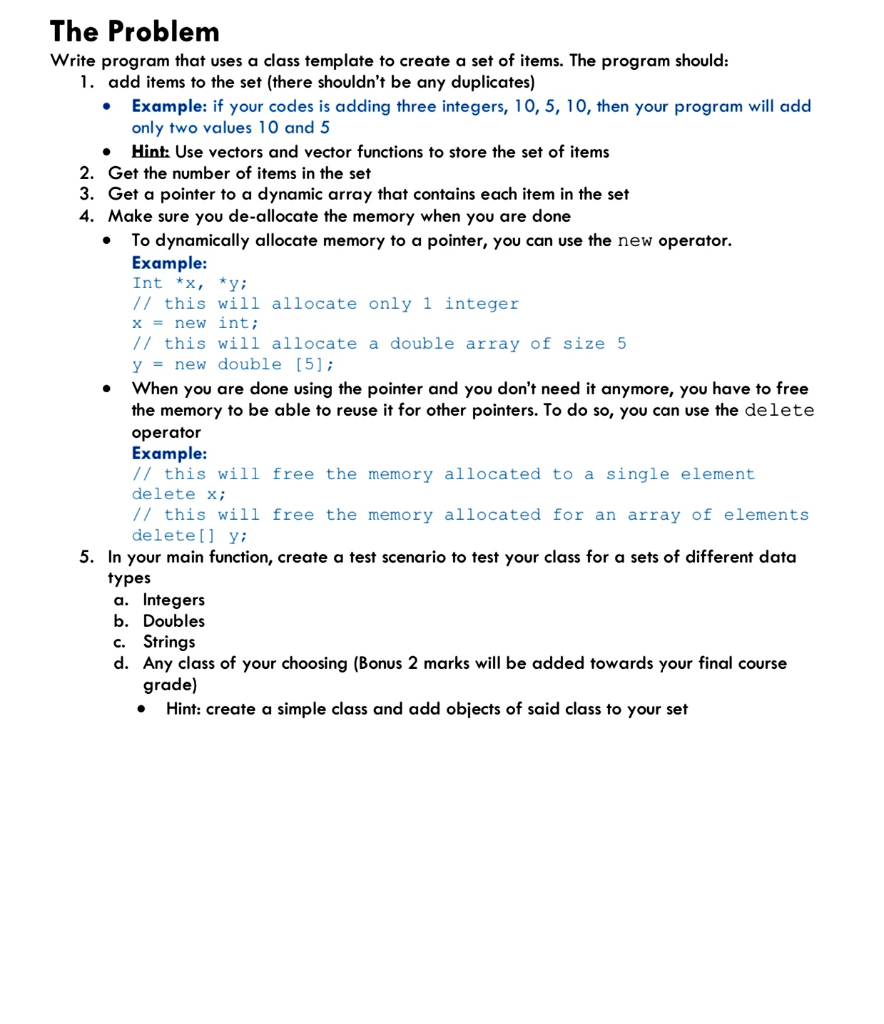Write a program in C++ that uses a class template to create a set of items. . . The Problem Write program that uses a class template to create a set of items. The program should: 1. add items to the set (there shouldn't be any duplicates) Example: if your codes is adding three integers, 10, 5, 10, then your program will add only two values 10 and 5 Hint: Use vectors and vector functions to store the set of...

• ### In C++ for 2D Array: Write a program that uses a function that manipulates a 2D...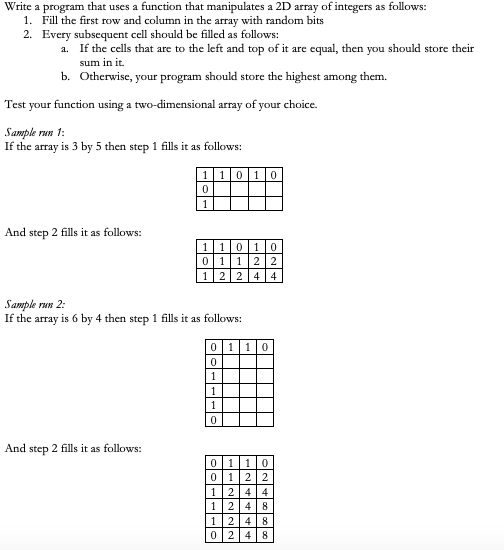In C++ for 2D Array: Write a program that uses a function that manipulates a 2D array of integers as follows: 1. Fill the first row and column in the array with random bits 2. Every subsequent cell should be filled as follows: 1. If the cells that are to the left and top of it are equal, then you should store their sum in it b. Otherwise, your program should store the highest among them. Test your function using...

• ### Hi please help with this program C++ language thanks Write a recursive solution to solve the...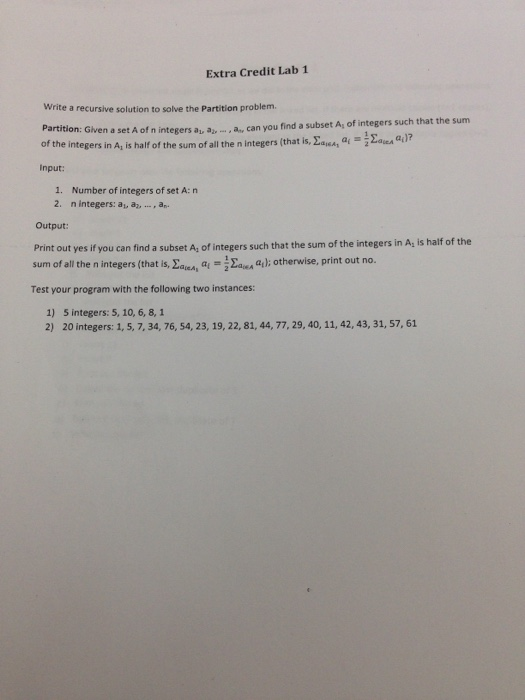Hi please help with this program C++ language thanks Write a recursive solution to solve the Partition problem. Partition: Given a set A of n integers a, a, .....a., can you find a subset A_1 of integers such that the sum of the Integers in A_1 is half of the Sum of all the n Integers (that Is, sigma_alpha 1 A_1 a_i)? Input: 1.Number of integers of set A: n 2.n Integers: a_1 a_2, a_n. Output: Print out yes if...

• ### Program Instructions: Write a C++ program that uses a random number generator to generate a two...

Program Instructions: Write a C++ program that uses a random number generator to generate a two digit positive integer and allows the user to perform one or more of the following operations: Double the number. Reverse the digits of the number. Raise the number to the power of 2, 3, or 4. Sum the digits of the number. If the number is a two digit number, then raise the first digit to the power of the second digit. If the...

• ### C++ please Question 4: 125 points] Write and test a program that reads two positive integers...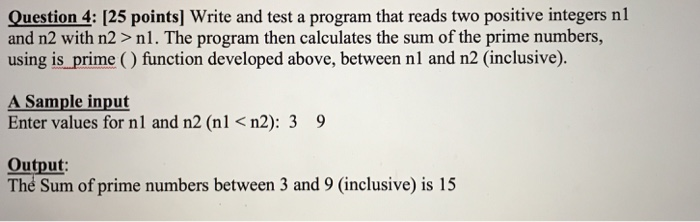C++ please Question 4: 125 points] Write and test a program that reads two positive integers nl and n2 with n2 > nl. The program then calculates the sum of the prime numbers, using is prime () function developed above, between nl and n2 (inclusive) A Sample input Enter values for nl and n2 (nl<n2): 3 9 Output: Thé Sum of prime numbers between 3 and 9 (inclusive) is 15